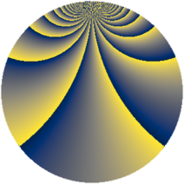# Properties

 Label 384.2.vLevel $384$ Weight $2$ Character orbit 384.v Rep. character $\chi_{384}(13,\cdot)$ Character field $\Q(\zeta_{32})$ Dimension $512$ Newform subspaces $1$ Sturm bound $128$ Trace bound $0$

# Related objects

## Defining parameters

 Level: $$N$$ $$=$$ $$384 = 2^{7} \cdot 3$$ Weight: $$k$$ $$=$$ $$2$$ Character orbit: $$[\chi]$$ $$=$$ 384.v (of order $$32$$ and degree $$16$$) Character conductor: $$\operatorname{cond}(\chi)$$ $$=$$ $$128$$ Character field: $$\Q(\zeta_{32})$$ Newform subspaces: $$1$$ Sturm bound: $$128$$ Trace bound: $$0$$

## Dimensions

The following table gives the dimensions of various subspaces of $$M_{2}(384, [\chi])$$.

Total New Old
Modular forms 1056 512 544
Cusp forms 992 512 480
Eisenstein series 64 0 64

## Trace form

 $$512 q + O(q^{10})$$ $$512 q - 96 q^{50} - 96 q^{52} - 32 q^{54} - 224 q^{56} - 192 q^{60} - 192 q^{62} - 192 q^{64} - 192 q^{66} - 192 q^{68} - 192 q^{70} - 224 q^{74} - 32 q^{76} - 96 q^{78} - 96 q^{80} + O(q^{100})$$

## Decomposition of $$S_{2}^{\mathrm{new}}(384, [\chi])$$ into newform subspaces

Label Dim $A$ Field CM Traces $q$-expansion
$a_{2}$ $a_{3}$ $a_{5}$ $a_{7}$
384.2.v.a $512$ $3.066$ None $$0$$ $$0$$ $$0$$ $$0$$

## Decomposition of $$S_{2}^{\mathrm{old}}(384, [\chi])$$ into lower level spaces

$$S_{2}^{\mathrm{old}}(384, [\chi]) \cong$$ $$S_{2}^{\mathrm{new}}(128, [\chi])$$$$^{\oplus 2}$$